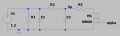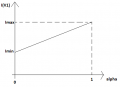# Duty cycle VS voltage problem

#### Oto

Joined Feb 22, 2017
5
Hello,

According to my schematic below, I would like to know the relation between the Duty Cycle (called alpha) of the NMOS gate, and the voltage Vp.
At the end, I want the relation between the current in the voltage source VS alpha, but from Vp, I will be able to find it easily.What I understand:
If C2 is removed from the circuit, it is easy to calculate the equivalent resistor made by R1, R2 and R3 according to alpha:
Req1 = R1*(1-alpha)+alpha*R1*(R2+R3)/(R1+R2+R3)
And then calculate the current in V1 :
I(V1) = V1/Req1

But the addition of C2 creates a non linearity and the above equation is no longer correct (because C2 is charged and discharged with a different time constant due to the difference between R2 and R3)

I tried to find the charging and discharging differential equations:
Vfinal_discharge =(Vfinal_charge-V1*R3/(R3+R2))*exp(-alpha*T/(C2*Req2))+V1*R3/(R3+R2)
Vfinal_charge == (V1-Vfinal_discharge)*(1-exp(-T*(1-alpha)/(C2*Req2)))+Vfinal_discharge
with :
T=switching period
Req2 = R2+R3 / (R2*R3)

Resolving them (2 equations and 2 unknowns) does not seam to give a correct relation...

If you have any idea, I would very much appreciate.

Thank you,
Oto

#### Papabravo

Joined Feb 24, 2006
17,318
C2 is never removed from the circuit. the only function of the MOSFET is to partially discharge it. It cannot however bring Vp to zero because the discharge is exponential and asymptotic to 0.
R1/C1 is a high pass shunt, and R2/C2 is a lowpass section. The cascade of these two sections will have a lowpass characteristic.
Based on alpha, the circuit will alternate between a lowpass characteristic and an exponential decay.

I have no idea what that will look like on a Bode plot. I'm not even sure that I am curious enough to do the simulation.

#### Ian0

Joined Aug 7, 2020
3,785
If V1 has zero output impedance (as Spice voltage sources often do) then R1 and C1 are pretty much irrelevant.
All the components are resistors and capacitors, so there is no possibilities of any non-linearity.
If C2 is large in comparison to the switching frequency, then the effective value of R3 is 1/alpha times its real value.

•Papabravo

#### Papabravo

Joined Feb 24, 2006
17,318
If V1 has zero output impedance (as Spice voltage sources often do) then R1 and C1 are pretty much irrelevant.
...
Good point. I missed that. Add an output impedance and do an AC analysis. I'm curious but not curious enough to do any actual work. (that's right..I've turned into a Choosing Beggar)

#### Oto

Joined Feb 22, 2017
5
Thank you for your replies !

C2 is never removed from the circuit. the only function of the MOSFET is to partially discharge it. It cannot however bring Vp to zero because the discharge is exponential and asymptotic to 0.
R1/C1 is a high pass shunt, and R2/C2 is a lowpass section. The cascade of these two sections will have a lowpass characteristic.
Based on alpha, the circuit will alternate between a lowpass characteristic and an exponential decay.

I have no idea what that will look like on a Bode plot. I'm not even sure that I am curious enough to do the simulation.
Of course C2 is never removed. But when you compare the circuit with and without C2, the transfert function of Alpha VS Vp changes (at stable state).

If V1 has zero output impedance (as Spice voltage sources often do) then R1 and C1 are pretty much irrelevant.
All the components are resistors and capacitors, so there is no possibilities of any non-linearity.
If C2 is large in comparison to the switching frequency, then the effective value of R3 is 1/alpha times its real value.
Yes R1 and C1 have no impact on the voltage, it just makes a current offset in the source voltage (not C1 of course)

Let me explain the main problem.
I made this circuit to get the current of V1 dependents of alpha. I wanted the following response:With:
Imax = V1 / ( R1*(R2+R3)/(R1+R2+R3) )
Imin = V1 / R1

With the addition of C2, the curve is no longer I = Ax + B (but the limits Imin and Imax are still the same)

I made a simulation to show you the difference between both circuits.

C2 is mandatory to filter the PWM and get a low ripple current source.
But now I would like to find the mathematical relation between alpha and I(V1), which is not quite simple finally....

#### Attachments

• 4 KB Views: 2

#### Ian0

Joined Aug 7, 2020
3,785
Assume that C2 is "large" as in the ripple voltage is insignificant.
Let I equal the average MOSFET current.
I=alpha*Vp/R3
also
I= (V1-Vp)/R2
Vp=V1-I.R2
so
Vp=V1-(alpha.Vp/R3)*R2
V1=Vp+Vp.alpha*(R2/R3)
V1=Vp.(1+alpha*(R2/R3))
Vp=V1/(1+alpha*(R2/R3))
so
I=(V1*alpha)/(R3*(1+alpha*(R2/R3)))

How about swapping R2 for an inductor? Then the current equation is much simpler.
R2 would be the series resistance of the inductor, which would be almost zero.

#### Oto

Joined Feb 22, 2017
5
Thank you lan0, it works !

Yes It could be an inductor, but the capacitive load at low frequency seen from the source must be close to the value of C1. Moreover, a resistor is cheaper than an inductor.

#### Ian0

Joined Aug 7, 2020
3,785
Thank you lan0, it works !

Yes It could be an inductor, but the capacitive load at low frequency seen from the source must be close to the value of C1. Moreover, a resistor is cheaper than an inductor.
and smaller!
Though the inductor will isolate C2 from the source more than a resistor would.
The concept of a reactive component being "large" or "small" so that is either short-circuit or open-circuit at the frequencies in question makes the maths easier at proof-of-concept stage.
If the concept looks viable, then you can go on to do a more thorough analysis.
Just occasionally, you assume something to be of no significance when, in fact, it is important!